## 多变量梯度下降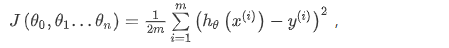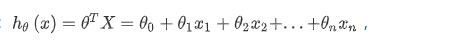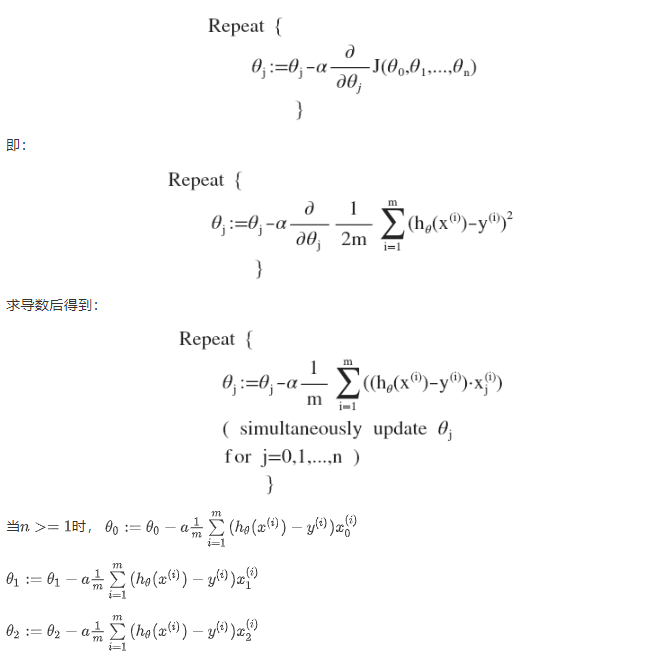Python 代码示例：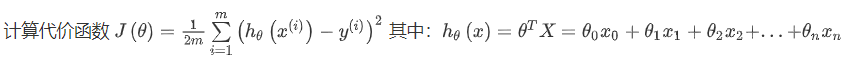``````def computeCost(X, y, theta):
inner = np.power(((X * theta.T) - y), 2)
return np.sum(inner) / (2 * len(X))
``````

## 梯度下降法实践1-特征缩放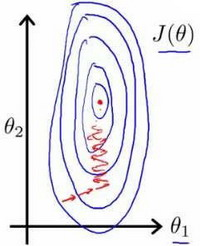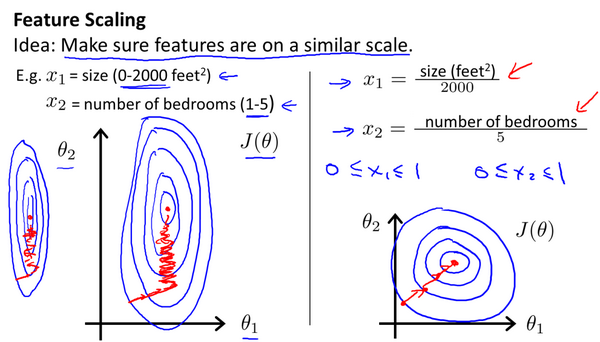## 梯度下降法实践2—学习率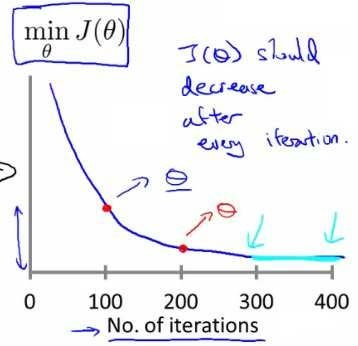`alpha = 0.01, 0.03, 0.1, 0.3, 1, 3, 10`

## 特征和多项式回归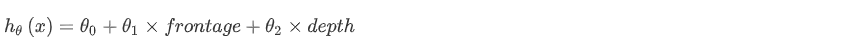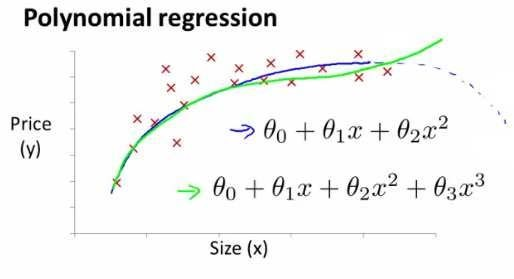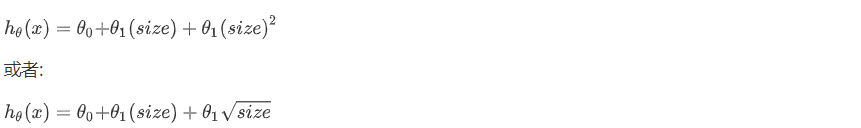## 正规方程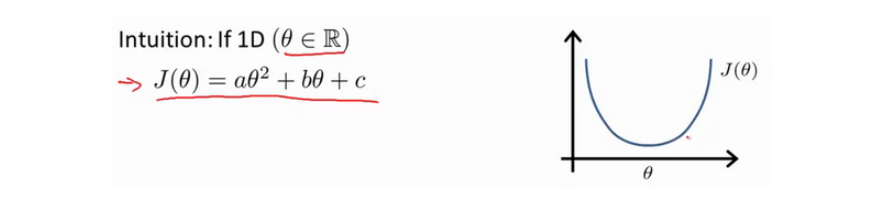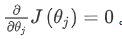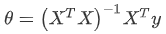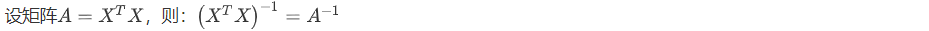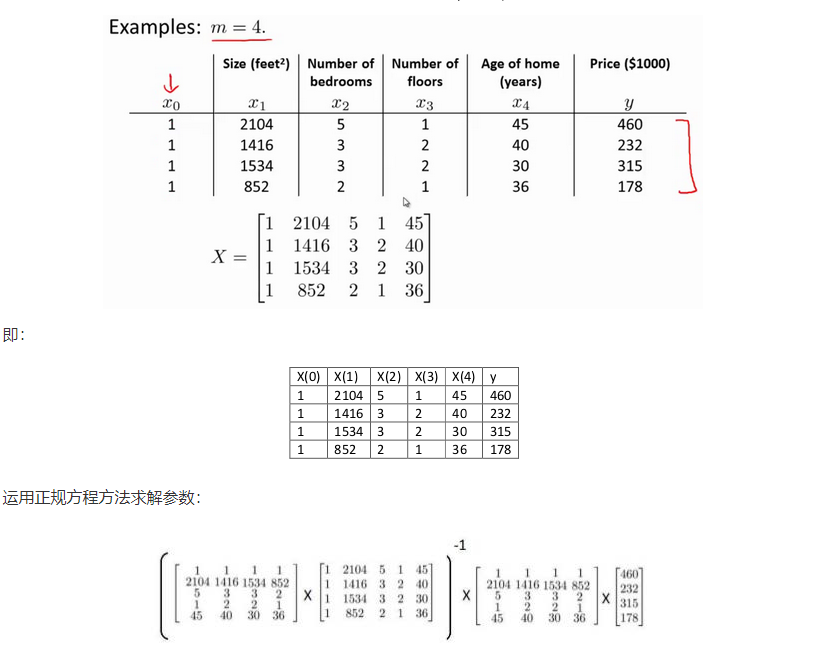``````import numpy as np

def normalEqn(X, y):
theta = np.linalg.inv(X.T@X)@X.T@y
#X.T@X等价于X.T.dot(X)
return theta
``````
Licensed under CC BY-NC-SA 4.0